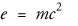## inlineequation

inlineequation — A mathematical equation or expression occurring inline.

## Synopsis

inlineequation ::=

None.

## Description

An `inlineequation`s is an expression (usually mathematical) that occurs in the text flow.

### Processing expectations

Formatted inline.

### Attributes

any attribute

Any attribute in any other explicit namespace

### Parents

These elements contain `inlineequation`: .

### Children

The following elements occur in `inlineequation`: .

Related elements: .

## Examples

``` 1 |`<article xmlns='http://docbook.org/ns/docbook'>`
|`<title>Example inlineequation</title>`
|` `
|`<para>Einstein's theory of relativity includes one of the most`
5 |`widely recognized formulas in the world:`
|`<inlineequation>`
|`  <alt>e=mc^2</alt>`
|`  <inlinemediaobject>`
|`    <imageobject condition="print">`
10 |`      <imagedata fileref="figs/print/db5d_refeqn03.pdf"/>`
|`    </imageobject>`
|`    <imageobject condition="web">`
|`      <imagedata fileref="figs/web/db5d_refeqn03.png"/>`
|`    </imageobject>`
15 |`  </inlinemediaobject>`
|`</inlineequation>`
|`</para>`
|` `
|`</article>`
```

Einstein's theory of relativity includes one of the most widely recognized formulas in the world: